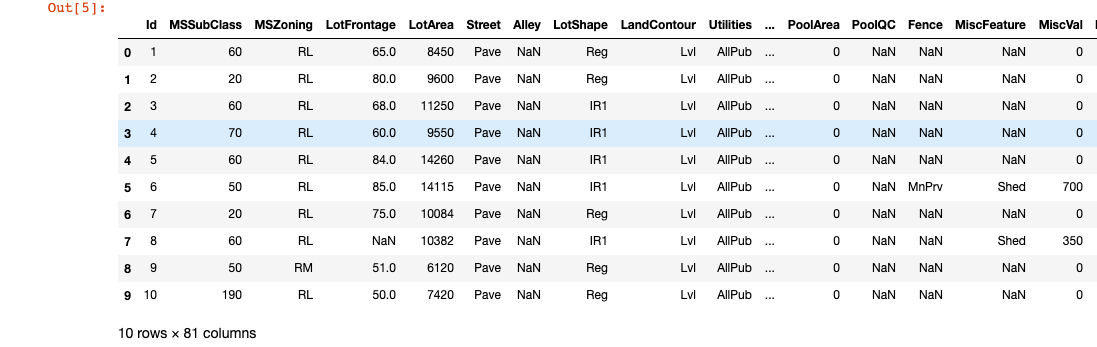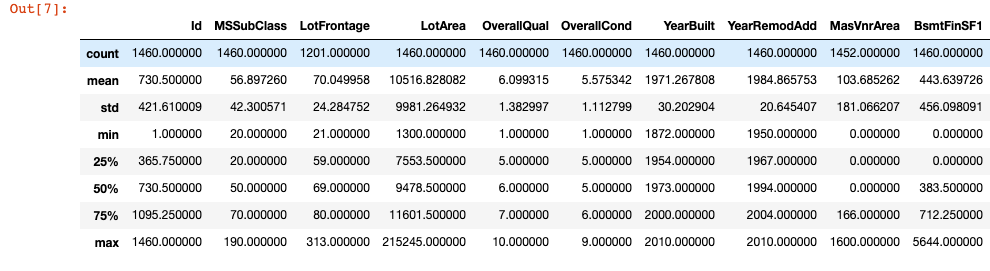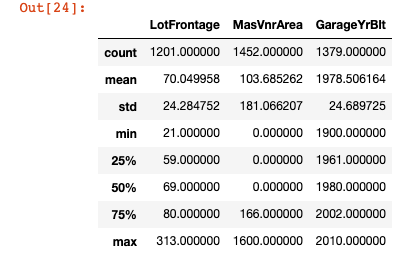# Pandas Dataframe Functions

### Learn the basics of Pandas' Dataframe.

· Big Data Zone · Tutorial
Save
8.50K Views

Pandas is a Python library that allows users to parse, clean, and visually represent data quickly and efficiently. Here, I will share some useful Dataframe functions that will help you analyze a data set.

First, you have to import the library. Conventionally, we use the alias, "pd," to refer to Pandas.

``import pandas as pd # data processing, CSV file I/O (e.g. pd.read_csv)``

The data, which is used in the example code, is taken from Kaggle House Prices. Specifically, I used the train.csv file. Save the file in the same folder with your code; otherwise, you have to give the path detail when reading the file.

``````#load data
df = pd.read_csv("train.csv")``````

Now that the data is loaded, we can the first and last nth number of rows in the dataframe using the head() and tail() methods, respectively.

``````#Just give an integer parameter as the number of rows
#Should be greater than zero.
#If you leave it blank, only first or last "5" rows will return.
df.head(10)   # First nth rows
df.tail(10)   # Last nth rows``````We can then use the describe() method in order to get some basic statistical information (row count, mean, standard deviation, quartiles, minimum, and maximum) about each column in our dataframe.

``df.describe()``

The output should look something like this:We can also use the transpose() method or .T in order to get a transposed version of our dataframe.

``````df.describe().T
df.describe().transpose()``````

The output will look something like this (for the first ten rows).You can also describe the columns according to their column data types as below;

``````print(df.select_dtypes(include=['int64','float64']).describe())
print(df.select_dtypes(include=['object']).describe())``````If you want to see each columns' name, number of rows, null-value, and data type, use the info() function. If you only want the data type, then use the dtypes attribute.

``````df.info()     # Get column name, number of rows, null, and data type.
df.dtypes     # get only data types``````

You can use this table later to define the numeric or non-numeric columns to handle some data manipulations on your data. This is especially useful for finding missing values.

You can use the size attribute of a Dataframe in order to get the total number of rows in each column.

``````# Returns size of dataframe/series which is equivalent to
#total number of elements. That is rows x columns.
df.size       ``````

Similarly, you can use the shape attribute in order to get a tuple of the row count and the column count. You can then index the tuple in order to isolate either of the values returned.

``````df.shape # Get a tuple of the row and column count
df.shape # Get just the row count
df.shape # Get just the column count``````

If you are working with Pandas object and can't determine if it's a Series or Dataframe object, you can use the ndim attribute. This returns the number of dimensions of the object (one if it is a Series, two if it is a Dataframe).

``````df.ndim       # Returns dimension of dataframe/series.
# 1 for one dimension (series), 2 for two dimension (dataframe) ``````

Every row has an index and an index value;

``````df.index          #index of rows -> Returns "RangeIndex(start=0, stop=1460, step=1)"
df.index.values   #index values of rows
df.index.tolist() #index``````

To get the distinct values of a column you can use the numpy library. Just as we alias Pandas to "pd", we also will follow the convention of aliasing the Numpy library as "np".

``````import numpy as np

print("Distinct Values for Overall Qualification&Condition")
overall_qual = np.unique(df['OverallQual'])
print(overall_qual)``````

You may want to get all the column names as a list and do some for loop calculations on them. This can be done by the following code;

``````all_columns_list = df.columns.tolist()
# get as a list of all the column names
for col in all_columns_list: print(col)
# just print the names, but you can do other jobs here``````
Topics:
pandas data frames tutorial, python

Opinions expressed by DZone contributors are their own.

Comments